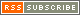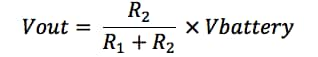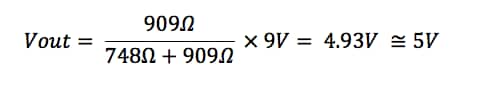India

 Indian Rupee Incoterms:FCA (Shipping Point) Duty, customs fees and taxes are collected at time of delivery. Payment accepted in Credit cards only

 US Dollars Incoterms:FCA (Shipping Point) Duty, customs fees and taxes are collected at time of delivery. All payment options available

••••••••••••••••••# Bench Talk### Bench Talk for Design Engineers | The Official Blog of Mouser Electronics

Applying The Basics: The Voltage DividerHello, everyone!  My series of posts are going to deal with applications of open source hardware.  My goals are to share applications using open source hardware and teach some fundamentals of electronics and software to turn these projects from concept to reality.  To make things easy as possible, I will put links to Mouser’s website so you can get parts ordered fast and get to making cool things sooner rather than later.

In the Arduino Yun-based package deliver notification project we utilized a voltage divider circuit with a force sensitive resistor to detect the weight of a package.  Having a variable resistor in a voltage divider allows an output voltage to change with respect to the change in resistance, which is in in response to a change in a physical stimulus.  Such changed could be a change in weight with the force sensitive resistor or temperature when using a thermistor.  Voltage dividers with two fixed resistors also useful for setting the DC bias voltage for bipolar junction transistor circuits.  Let’s take a deeper look at how a voltage divider works.  First, let’s look at a voltage divider circuit.

When you are prototyping your project we start with through-hole carbon film resistors since they inexpensive and breadboard compatible.    For the DIYer, the three important specifications of a resistor are the resistance value, the power (wattage) rating, and tolerance.

Simply put, a voltage divider takes an input voltage and drops it by an amount that is proportional to the ratio of the two resistors that makeup the divider network.   The output voltage, if you measure voltage across R2 is given by the following equation:Let’s talk a moment about how to decide the resistor values you will need for your voltage divider.  To do so, you must take into account the load that will be connected to the output of the voltage divider.  Let’s assume our load is 1KΩ and requires 5V at 5mA.  We now apply the “10% Rule” so that the “bleeder current” through R2 is 10% of the current that we want to follow though the load.  In this case:To calculate the value or R2 we calculate the following:Next we calculate the value for R1.We can get closer to the calculated value by adding a 68Ω in series to the 680Ω resistor resulting in equivalent resistance of 748Ω.

Let’s put all this together now and see if everything works out.  First we have to make a slight modification to the first equation to take an account the series resistors and the loading on the circuit.Where Req = R2 || Rload  therefore Req = 909Ω

and Rseries = 680Ω+68Ω = 748Ω

Now apply those values to Equation 5.Lastly, just make sure to calculate the power rating required for each resistor using the formula P = I2R.  We will cover that in further detail in a coming blog.  So there you have it, the voltage divider.  A simple but useful circuit used in a great many applications we will be discussing.

« BackMichael Parks, P.E. is the owner of Green Shoe Garage, a custom electronics design studio and technology consultancy located in Southern Maryland. He produces the S.T.E.A.M. Power podcast to help raise public awareness of technical and scientific matters. Michael is also a licensed Professional Engineer in the state of Maryland and holds a Master’s degree in systems engineering from Johns Hopkins University.